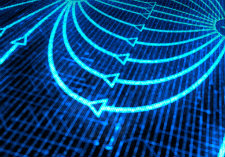AP Physics C Electricity and Magnetism

This course is equivalent to a first semester calculus-based college physics class for majors in the physical sciences, engineering, mathematics, or computational sciences. It is expected that students in this course will have a command of mathematical skills for basic differential and integral calculus, as these concepts are used throughout the course.

Course requirements: Since this is a calculus-based physics course, students are required to take Calculus AB concurrently as a minimum requisite. However, many students have already had Calculus AB upon entering the course and take Calculus BC concurrently. Students are expected to solve problems with a high degree of complexity consistent with a university-level electricity and magnetism syllabus.

Topics covered include measurement, electric forces, electric fields, magnetism, electromagnetism, electricity and circuits. Emphasis is placed on the use of algebra, trigonometry and calculus to solve problems and analyze data. Prior skills needed to achieve proficiency therefore include those learned in algebra, trigonometry, and calculus. Strong math and problem solving skills are therefore a necessity.

Units of Study

✓ Electric Charge
✓ Electric Fields
✓ Gauss’ Law
✓ Electric Potential
✓ Capacitance
✓ Current & Resistance
✓ Circuits
✓ Magnetic Fields
✓ Magnetic Fields due to Currents (Ampere’s Law)
✓ Induction and Inductance
✓ Magnetism of Matter; Maxwell’s Equations